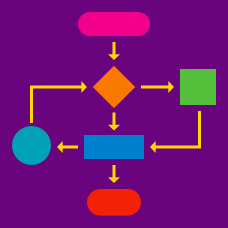Computer Science

# Beginner Algorithms Warmup

Suppose an algorithm with an input of the list: hand, eye, nose, face.

Step 1. Swap places with the 1st and 4th items on the list.

Step 2. Swap places with the 1st and 2nd items on the list.

Step 3. End.

What will be the list at Step 3?

Step 1. Set $\text{Loop} = 0$ and $\text{Doubler} = 1.$

Step 2. Add 1 to $\text{Loop} .$

Step 3. Multiply $\text{Doubler}$ by two.

Step 4. If $\text{Loop} < 4$ go to Step 2.

Step 5. End.

What is the value of $\text{Doubler}$ at Step 5?

Step 1. Set $\text{Loop} = 0$ and $\text{Multiple} = 0.$

Step 2. Add 1 to $\text{Loop} .$

Step 3. Add 3 to $\text{Multiple} .$

Step 4. If $\text{Loop} < 100$ go to Step 2.

Step 5. End.

What is the value of $\text{Multiple}$ at Step 5?

Suppose a function with an input of the string "ABCDEFG". (A is in the 1st position, B is in the 2nd position, and so on.)

Step 1. Set $\text{Loop} = 0 .$

Step 2. Add 1 to $\text{Loop} .$

Step 3. Swap the letter at position $\text{Loop}$ with the letter at position $\text{Loop} + 2 .$

Step 4. If $\text{Loop} < 4$ go to Step 2.

Step 5. End.

What will be the string at Step 5?

Suppose an algorithm with an input of the list: Apple, Banana, Carrot, Dragons.

Step 1. If the 1st item on the list has fewer letters than the 2nd item, swap the items.

Step 2. If the 2nd item on the list has fewer letters than the 4th item, swap the items.

Step 3. If the 2nd item on the list has fewer letters than the 3rd item, swap the items.

Step 4. End.

What will be the list at Step 4?

×